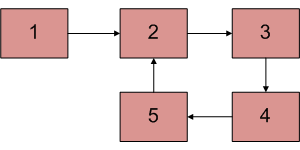# Java Program For Finding The Length Of Loop In Linked List

• Last Updated : 05 Apr, 2022

Write a function detectAndCountLoop() that checks whether a given Linked List contains loop and if loop is present then returns count of nodes in loop. For example, the loop is present in below-linked list and length of the loop is 4. If the loop is not present, then the function should return 0.Approach:
It is known that Floyd’s Cycle detection algorithm terminates when fast and slow pointers meet at a common point. It is also known that this common point is one of the loop nodes. Store the address of this common point in a pointer variable say (ptr). Then initialize a counter with 1 and start from the common point and keeps on visiting the next node and increasing the counter till the common pointer is reached again.
At that point, the value of the counter will be equal to the length of the loop.
Algorithm:

1. Find the common point in the loop by using the Floyd’s Cycle detection algorithm
2. Store the pointer in a temporary variable and keep a count = 0
3. Traverse the linked list until the same node is reached again and increase the count while moving to next node.
4. Print the count as length of loop

## Java

 `// Java program to count number of nodes ` `// in loop in a linked list if loop is ` `// present ` `import` `java.io.*;`   `class` `GFG ` `{` `    ``// Link list node` `    ``static` `class` `Node ` `    ``{ ` `        ``int` `data;` `        ``Node next;` `        ``Node(``int` `data)` `        ``{` `            ``this``.data =data;` `            ``next =``null``;` `        ``}` `    ``}`   `    ``// Returns count of nodes present ` `    ``// in loop. ` `    ``static` `int` `countNodes( Node n) ` `    ``{ ` `        ``int` `res = ``1``; ` `        ``Node temp = n; ` `        ``while` `(temp.next != n) ` `        ``{ ` `            ``res++; ` `            ``temp = temp.next; ` `        ``} ` `        ``return` `res; ` `    ``} `   `    ``/* This function detects and counts ` `       ``loop nodes in the list. If loop ` `       ``is not there in then returns 0 */` `    ``static` `int` `countNodesinLoop( Node list) ` `    ``{ ` `        ``Node slow_p = list, ` `             ``fast_p = list; `   `        ``while` `(slow_p !=``null` `&& ` `               ``fast_p!=``null` `&& ` `               ``fast_p.next!=``null``) ` `        ``{ ` `            ``slow_p = slow_p.next; ` `            ``fast_p = fast_p.next.next; `   `            ``/* If slow_p and fast_p meet at some ` `               ``point then there is a loop */` `            ``if` `(slow_p == fast_p) ` `                ``return` `countNodes(slow_p); ` `        ``} `   `        ``// Return 0 to indicate that there is ` `        ``// no loop` `        ``return` `0``; ` `    ``} `   `    ``static` `Node newNode(``int` `key) ` `    ``{ ` `        ``Node temp = ``new` `Node(key);    ` `        ``return` `temp; ` `    ``} `   `    ``// Driver code` `    ``public` `static` `void` `main (String[] args) ` `    ``{` `        ``Node head = newNode(``1``); ` `        ``head.next = newNode(``2``); ` `        ``head.next.next = newNode(``3``); ` `        ``head.next.next.next = newNode(``4``); ` `        ``head.next.next.next.next = newNode(``5``); `   `        ``// Create a loop for testing ` `        ``head.next.next.next.next.next = head.next; `   `        ``System.out.println( countNodesinLoop(head)); ` `    ``}` `}` `// This code is contributed by inder_verma.`

Output:

`4`

Complexity Analysis:

• Time complexity:O(n).
Only one traversal of the linked list is needed.
• Auxiliary Space:O(1).
As no extra space is required.

Please refer complete article on Find length of loop in linked list for more details!

My Personal Notes arrow_drop_up
Recommended Articles
Page :